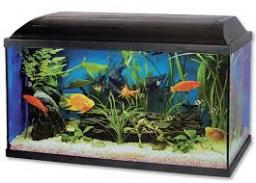# Dimensions 6130

The aquarium dimensions are in the ratio a: b: c = 5: 2: 4. 6609 cm2 of glass was used for its production. How many liters of water will fit in the aquarium if it reaches 5 cm below its edge?

V =  35.075 l

### Step-by-step explanation:Did you find an error or inaccuracy? Feel free to write us. Thank you!

Tips for related online calculators
Are you looking for help with calculating roots of a quadratic equation?
Do you have a linear equation or system of equations and looking for its solution? Or do you have a quadratic equation?
Do you know the volume and unit volume, and want to convert volume units?

#### Grade of the word problem:

We encourage you to watch this tutorial video on this math problem: